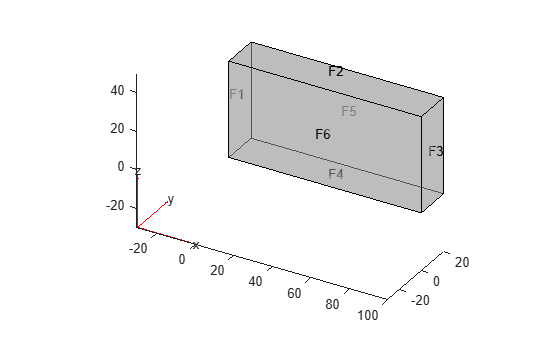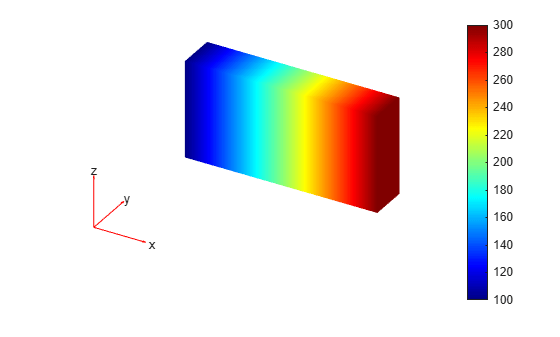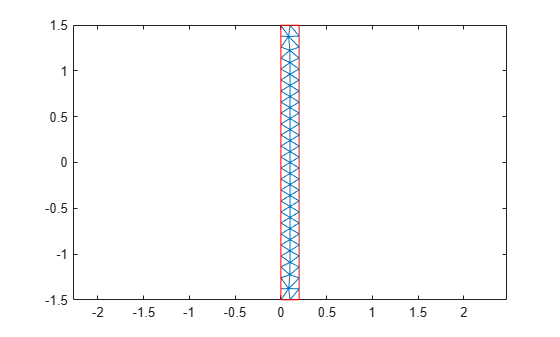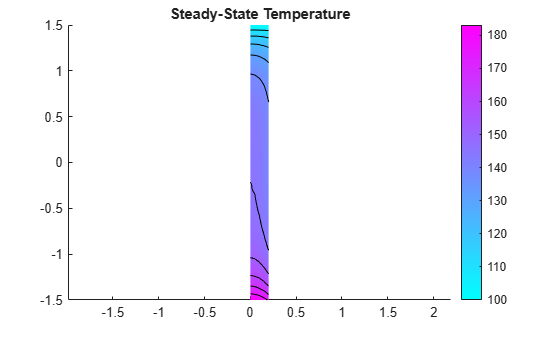Steady-state thermal solution and derived quantities

## Description

A `SteadyStateThermalResults` object contains the temperature and temperature gradient values in a form convenient for plotting and postprocessing.

The temperature and its gradients are calculated at the nodes of the triangular or tetrahedral mesh generated by `generateMesh`. Temperature values at the nodes appear in the `Temperature` property. The three components of the temperature gradient at the nodes appear in the `XGradients`, `YGradients`, and `ZGradients` properties.

To interpolate the temperature or its gradients to a custom grid (for example, specified by `meshgrid`), use `interpolateTemperature` or `evaluateTemperatureGradient`.

To evaluate heat flux of a thermal solution at nodal or arbitrary spatial locations, use `evaluateHeatFlux`. To evaluate integrated heat flow rate normal to a specified boundary, use `evaluateHeatRate`.

## Creation

Solve a steady-state thermal problem using the `solve` function. This function returns a steady-state thermal solution as a `SteadyStateThermalResults` object.

## Properties

expand all

Finite element mesh, returned as an FEMesh Properties object.

Temperature values at nodes, returned as a vector.

Data Types: `double`

x-component of the temperature gradient at nodes, returned as a vector.

Data Types: `double`

y-component of the temperature gradient at nodes, returned as a vector.

Data Types: `double`

z-component of the temperature gradient at nodes, returned as a vector.

Data Types: `double`

r-component of the temperature gradient at nodes, returned as a vector.

Data Types: `double`

z-component of the temperature gradient at nodes, returned as a vector.

Data Types: `double`

## Object Functions

 `evaluateHeatFlux` Evaluate heat flux of a thermal solution at nodal or arbitrary spatial locations `evaluateHeatRate` Evaluate integrated heat flow rate normal to specified boundary `evaluateTemperatureGradient` Evaluate temperature gradient of a thermal solution at arbitrary spatial locations `interpolateTemperature` Interpolate temperature in a thermal result at arbitrary spatial locations

## Examples

collapse all

Solve a 3-D steady-state thermal problem.

Create a thermal model for this problem.

`thermalmodel = createpde('thermal');`

Import and plot the block geometry.

```importGeometry(thermalmodel,'Block.stl'); pdegplot(thermalmodel,'FaceLabel','on','FaceAlpha',0.5) axis equal```Assign material properties.

`thermalProperties(thermalmodel,'ThermalConductivity',80);`

Apply a constant temperature of 100 °C to the left side of the block (face 1) and a constant temperature of 300 °C to the right side of the block (face 3). All other faces are insulated by default.

```thermalBC(thermalmodel,'Face',1,'Temperature',100); thermalBC(thermalmodel,'Face',3,'Temperature',300);```

Mesh the geometry and solve the problem.

```generateMesh(thermalmodel); thermalresults = solve(thermalmodel)```
```thermalresults = SteadyStateThermalResults with properties: Temperature: [12691x1 double] XGradients: [12691x1 double] YGradients: [12691x1 double] ZGradients: [12691x1 double] Mesh: [1x1 FEMesh] ```

The solver finds the temperatures and temperature gradients at the nodal locations. To access these values, use `thermalresults.Temperature`, `thermalresults.XGradients`, and so on. For example, plot temperatures at the nodal locations.

`pdeplot3D(thermalmodel,'ColorMapData',thermalresults.Temperature)`Analyze heat transfer in a rod with a circular cross-section and internal heat generation by simplifying a 3-D axisymmetric model to a 2-D model.

Create a steady-state thermal model for solving an axisymmetric problem.

`thermalmodel = createpde('thermal','steadystate-axisymmetric');`

The 2-D model is a rectangular strip whose x-dimension extends from the axis of symmetry to the outer surface and whose y-dimension extends over the actual length of the rod (from `-`1.5 m to 1.5 m). Create the geometry by specifying the coordinates of its four corners. For axisymmetric models, the toolbox assumes that the axis of rotation is the vertical axis passing through r = 0.

`g = decsg([3 4 0 0 .2 .2 -1.5 1.5 1.5 -1.5]');`

Include the geometry in the model.

`geometryFromEdges(thermalmodel,g);`

Plot the geometry with the edge labels.

```figure pdegplot(thermalmodel,'EdgeLabels','on') axis equal```The rod is composed of a material with these thermal properties.

```k = 40; % thermal conductivity, W/(m*C) q = 20000; % heat source, W/m^3```

For a steady-state analysis, specify the thermal conductivity of the material.

`thermalProperties(thermalmodel,'ThermalConductivity',k);`

Specify the internal heat source.

`internalHeatSource(thermalmodel,q);`

Define the boundary conditions. There is no heat transferred in the direction normal to the axis of symmetry (edge 1). You do not need to change the default boundary condition for this edge. Edge 2 is kept at a constant temperature T = 100 °C.

`thermalBC(thermalmodel,'Edge',2,'Temperature',100);`

Specify the convection boundary condition on the outer boundary (edge 3). The surrounding temperature at the outer boundary is 100 °C, and the heat transfer coefficient is $50\text{\hspace{0.17em}}\mathrm{W}/\left(\mathrm{m}{\cdot }^{\circ }\mathrm{C}\right)$.

```thermalBC(thermalmodel,'Edge',3,... 'ConvectionCoefficient',50,... 'AmbientTemperature',100);```

The heat flux at the bottom of the rod (edge 4) is $5000\text{\hspace{0.17em}}\mathrm{W}/{\mathrm{m}}^{2}$.

`thermalBC(thermalmodel,'Edge',4,'HeatFlux',5000);`

Generate the mesh.

```msh = generateMesh(thermalmodel); figure pdeplot(thermalmodel) axis equal```Solve the problem.

`thermalresults = solve(thermalmodel)`
```thermalresults = SteadyStateThermalResults with properties: Temperature: [259x1 double] RGradients: [259x1 double] ZGradients: [259x1 double] Mesh: [1x1 FEMesh] ```

The solver finds the temperatures and temperature gradients at the nodal locations. To access these values, use `thermalresults.Temperature`, `thermalresults.RGradients`, and `thermalresults.ZGradients`. For example, plot temperatures at the nodal locations.

```T = thermalresults.Temperature; figure pdeplot(thermalmodel,'XYData',T,'Contour','on') axis equal title 'Steady-State Temperature'```Introduced in R2017a

## SupportGet trial now